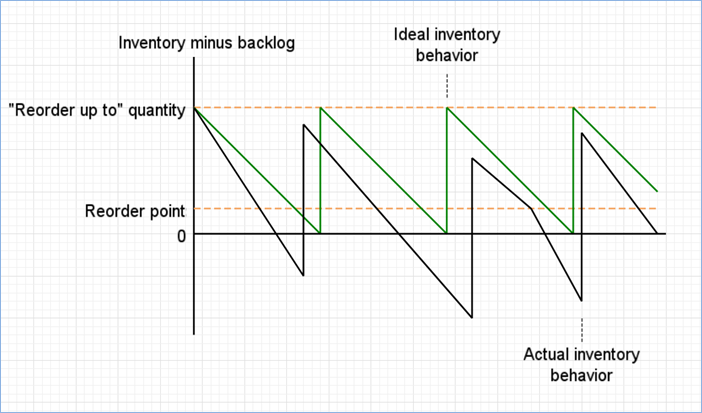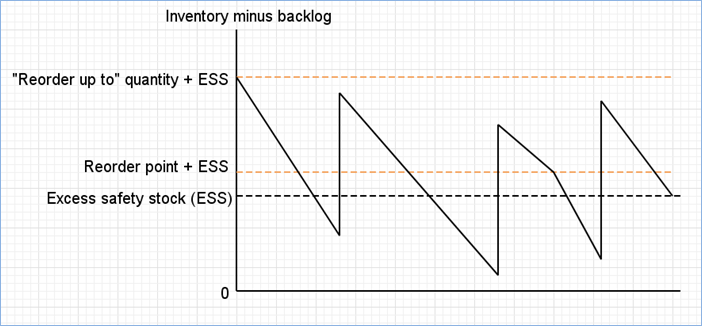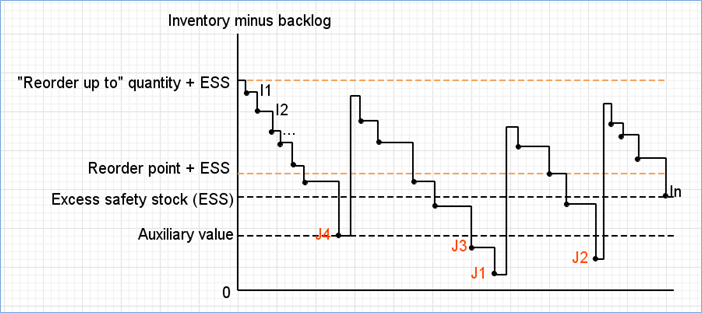anyLogistix

# How it works

Safety stock value can be estimated by the Safety Stock experiment, which is used to maintain the desirable service level within your supply chain. For details on the parameters of the experiment and the results it produces, refer here and here respectively.

The received safety stock value is used to either modify the existing inventory policy or create a new one that will eliminate delay or loss of products.

The inventory policies that are not available in the Safety stock estimation experiment:

• Unlimited inventory
• Order on demand
• Regular policy
• No replenishment
• Cross Dock policy

Let us see how it works.

The image below shows a simple example of a DC inventory within our supply chain, and the way it changes over time:The Inventory minus backlog stays less than 0 for a significant period of time, that is why we need certain safety stock to be able to provide the required service level.

Estimating safety stock value

1. anyLogistix adds excess safety stock value to the initial stock, the reordering point, the “reorder up to” quantity, and runs the simulation. The excess safety stock is just a number, which is knowingly large enough to avoid inventory stock outs. The exact value does not matter. This gives us the following dynamics:In the current simple example, the shape of the actual inventory behavior chart has not changed, but it changes in most cases if no stock outs happen, because all orders in this case are sent immediately and spend no time waiting for products.

The inventory behavior is based on two processes:

• Orders coming from downstream objects decrease inventory.
• Replenishment shipments arriving from upstream objects increase inventory.

Though it may look smooth from the whole simulation duration perspective, it is a step function by nature, i.e., all changes happen in particular moments of time. It actually looks like this:2. Let us denote each new inventory value after an order is sent as I1, I2, .. In (see the figure above) where n is the total number of orders.
Assume we want to keep the service level measured by product units equal to 95%. Let us denote the total sum of orders as S.
3. Our goal is to find the Auxiliary value (AV), which will be the smallest number satisfying the condition, where the sum of all orders (leading to inventory dropping below the AV) is greater than or equal to 5% of S. To do that we order increasingly {I1, .. In} i.

Assume we get an arranged set {J1, .. Jn} where a previous element is less than or equal to the next one. Then we will take an order corresponding to J1, and assume that its amount of product units is equal to O_j1. If O_j1 > 0.05*S, then J1 is the necessary value. If it is not, the next J2 value must be taken with the corresponding O_j2 order size to test if O_j1 + O_j2 > 0.05*S. If the condition is met, the J2 is what we need.

4. This calculation must be repeated until the necessary value is found or Jn is reached. In the latter case Jn is the necessary value. The auxiliary value corresponds to the expected level of 0, which will allow us to reach the required service level. Having found the AV, we may calculate the required safety stock value:
safety stock = max(0,excess safety stock — auxiliary value)